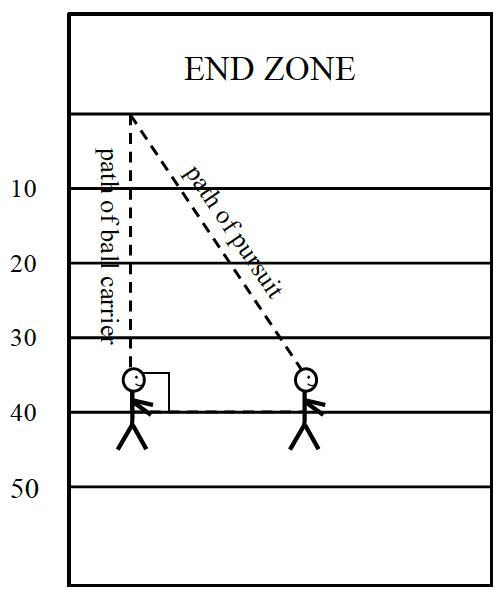### Home > GB8I > Chapter 4 Unit 5 > Lesson INT1: 4.1.1 > Problem4-6

4-6.

In football, the path that a defender must run to tackle the ball carrier is called the path of pursuit. If the ball carrier runs $40$ yards to the end zone and the path of pursuit is $45$ yards, how far apart were the ball carrier and defender when they started? Answer with appropriate precision. (Hint: Use the Pythagorean Theorem. If you need help, refer to the Math Notes box in this lesson.) Homework Help ✎

Which side of the formed triangle is the hypotenuse?

The path of pursuit is the hypotenuse.